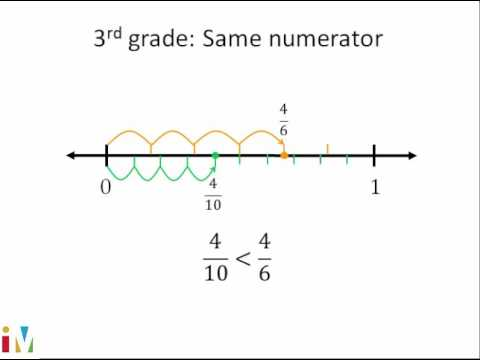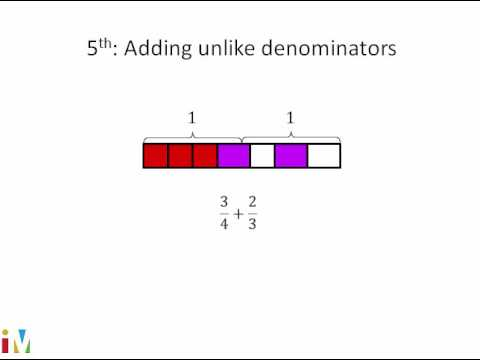# Visually dividing whole numbers by unit fractions (Full video)

Description: This video uses a number line and a tape diagram to explain 5 ÷ 1/2. If five is divided into pieces that are each one half of a whole, how many pieces are there? How many pieces would you get if you divide five into pieces that are each one half of a whole? So here on this number line we go from zero to five, and then notice they've divided into pieces that are each a half of a whole.

### Other videos you might be interested in### Comparing Fractions

#### Illustrative Mathematics